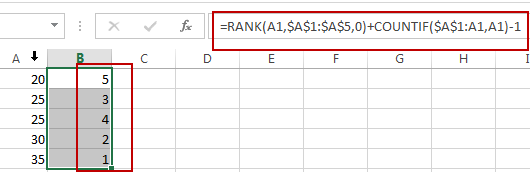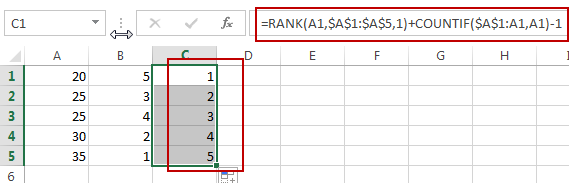# How to Rank Numbers without Repetitive Ranks in Excel

This post will guide you how to rank range numbers with unique ranking in Excel. How do I use Rank function to get the unique ranking of values for a range with duplicate values in Excel 2013/ 2016.

## Ranking without Repetitive Ranks

Assuming that you try to use the RANK function to sort values in a range of cells with two or more duplicate values. And the RANK function will return the same ranking of values for some duplicate values.

For example, if I have a list of data contain number values in column A (10,12,12,14,16), it return the ranking of values as 5,4,4,2,1 in another column. So the returned result is not your expected. And how do I get the results as: 5,4,3,2,1 for this example. You need to use a formula based on RANK function in combination with COUNTIF function to achieve the result of getting the unique ranking values.

Here is a formula that used to sort numbers with unique ranking:

`=RANK(A1,\$A\$1:\$A\$5,0)+COUNTIF(\$A\$1:A1,A1)-1`

Type this formula in Cell B1, and press Enter key on your keyboard, and then drag the Fill Handle down till B5.You would notice that the unique ranking numbers are calculated in descending order.

If you want to get the unique ranking values in ascending order for a range of cells, you can use another formula based on the RANK function and the COUNTIF function. Like this:

`=RANK(A1,\$A\$1:\$A\$5,1)+COUNTIF(\$A\$1:A1,A1)-1`

Type this formula in Cell C1, and press Enter key on your keyboard, and then drag the Fill Handle down till C5.### Related Functions

• Excel COUNTIF function
The Excel COUNTIF function will count the number of cells in a range that meet a given criteria. This function can be used to count the different kinds of cells with number, date, text values, blank, non-blanks, or containing specific characters.etc.= COUNTIF (range, criteria)…
• Excel Rank function
The Excel RANK function returns the rank of a given number in a supplied range of cells.  If there are duplicated values in a list of values, it will be set the same rank.The syntax of the RANK function is as below:= RANK (number,ref,[order])….

### You might also like:

Sidebar